### Modal analysis of transonic shock buffets on a NACA-0012 airfoil

Andre Weiner, Richard Semaan
TU Braunschweig, Institute of Fluid Mechanics

## Outline

1. Investigating shock buffets on airfoils
2. Simulation approach and sampling
3. Dynamic mode decomposition overview
4. Test of state vectors and DMD variants
5. Selected results

Slice of local Mach number $Ma$; $Re_\infty=10^7$, $Ma_\infty=0.75$, $\alpha=4^\circ$.

FOR 2895

Unsteady flow and interaction phenomena at high speed stall conditions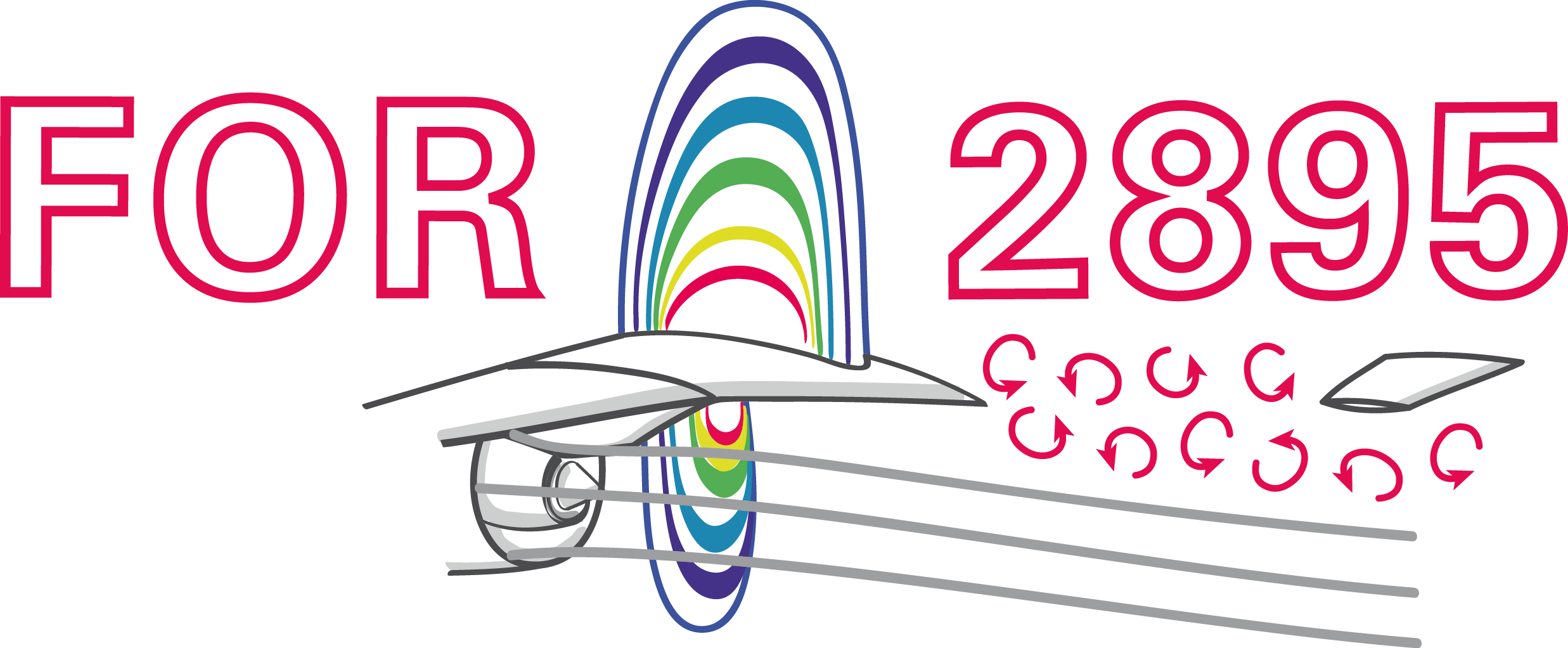Improved understanding and prediction of flight envelop via:

• experiments: iPSP, PIV, Schlieren, ...
• simulations: LES, RANS, hybrid RANS/LES
• analysis and modeling: modal decomposition, ROMs

Modal decomp. = spatial structures + temp. behavior

Common issues/questions in DMD analyses:

• How to construct the state vector?
• Which DMD variant to use?
• How much data is needed?
• How to set the rank parameter?
• How sensitive to noise is the analysis?

$\rightarrow$ (partial) answers in this presentation

## Simulation approach

Simulation approach in a nutshell:

• OpenFOAM-v2012
• rhoCentralFoam
• IDDES turbulence modeling
• Spalart-Allmaras closure
• 2D and 3D simulations

github.com/FlowModelingControl/naca0012_shock_buffet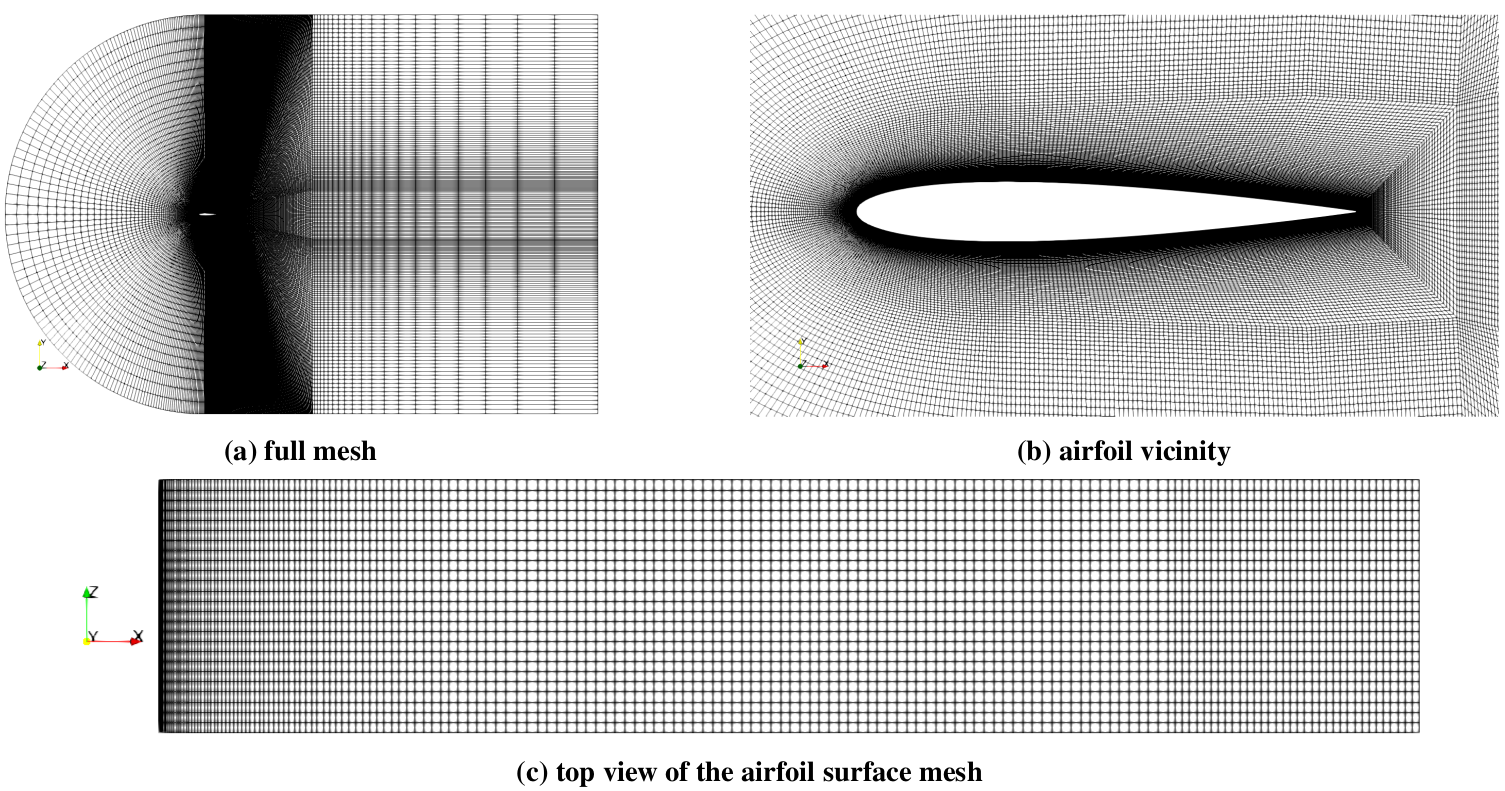Various views of the computational mesh.

## Dynamic mode decomposition

Degrees of freedom in DMD analysis:

1. State vector definition
2. Data pre-processing
3. Operator approximation
4. Mode amplitude computation
5. Mode selection

Review of DMD variants by P. Schmid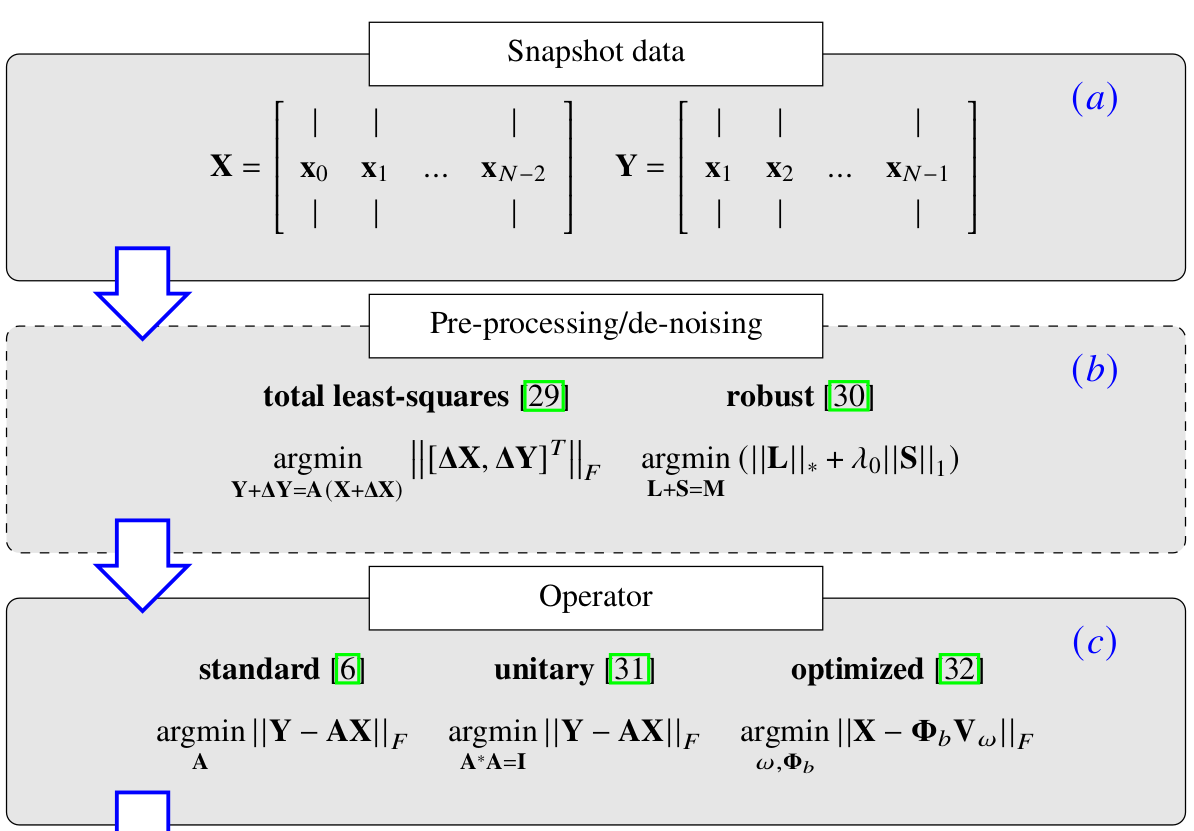DMD as a five-step analysis - part I.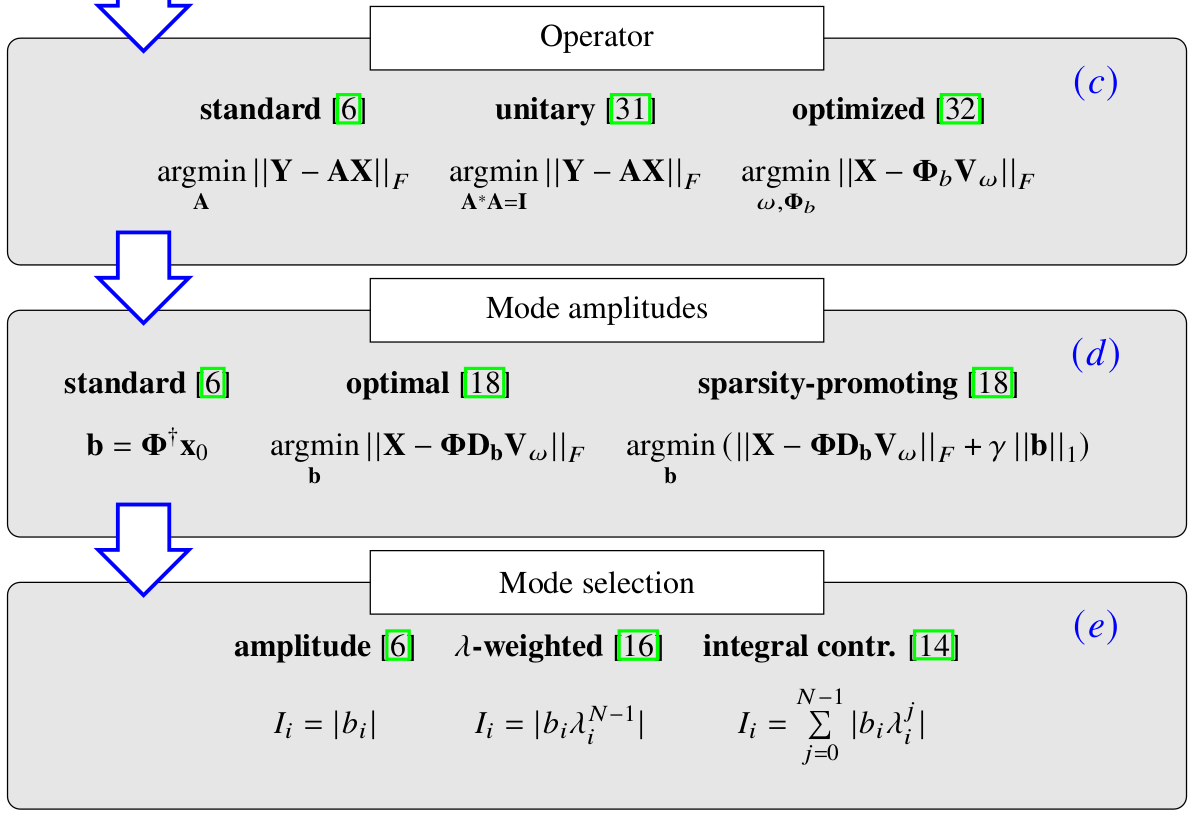DMD as a five-step analysis - part II.

Abbreviations:

• TDMD - total least squares DMD
• UDMD - unitary DMD operator
• opt. - optimal mode coefficients $\mathbf{b}$
• int. - integral importance measure
• amp. - classical DMD amplitude $|b_i|$

opt. TDMD, int. $\rightarrow$ total least-squares DMD with optimal amplitudes and integral mode selection.

## Test of state vectors and DMD variants

Test 0: variance of state vector normsTest 1: rank sensitivity of buffet frequency

Assumption: top mode = buffet mode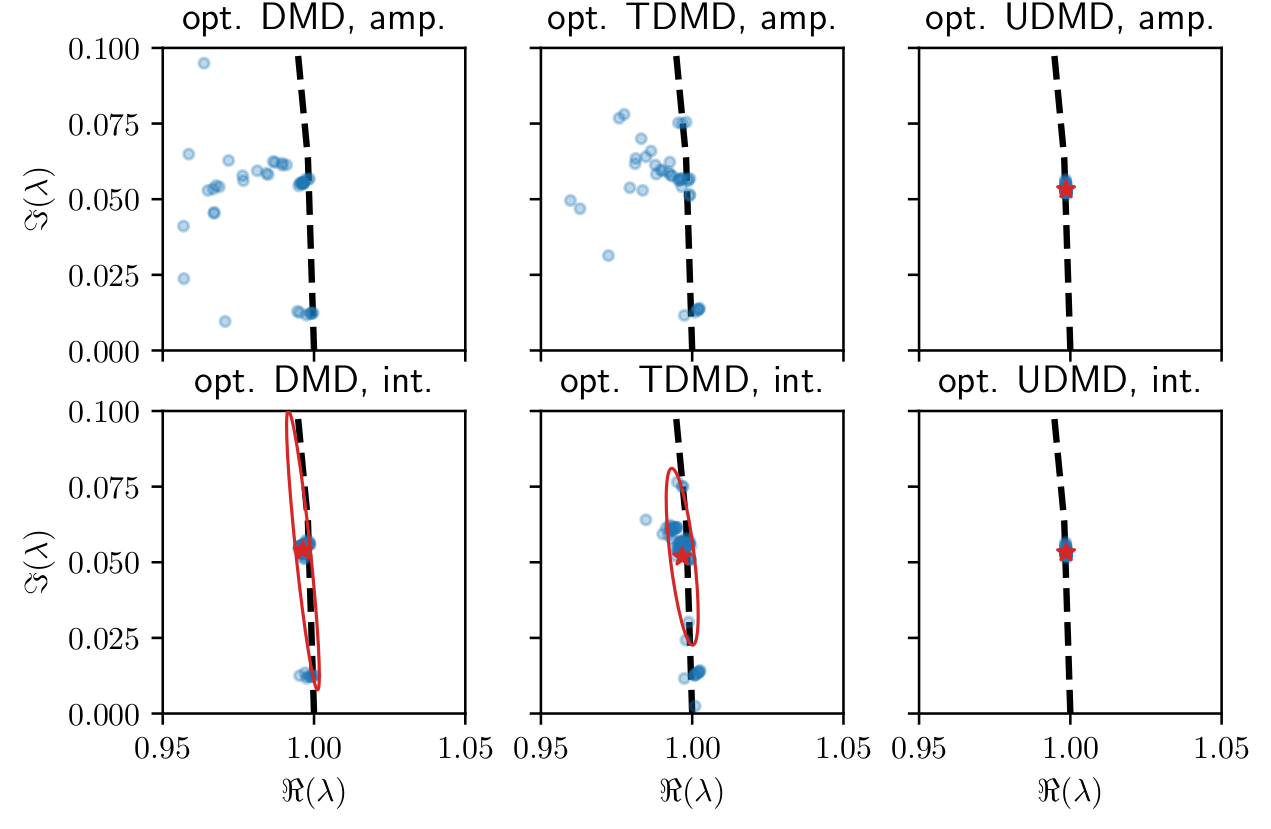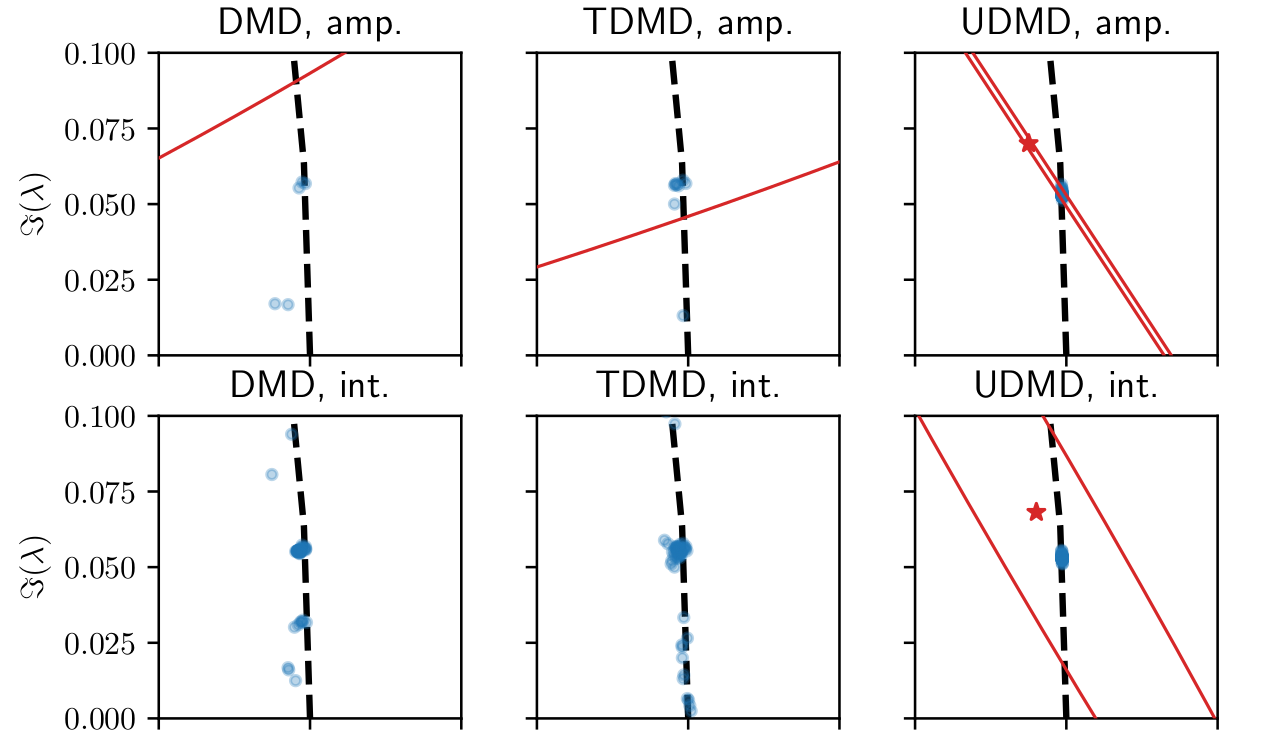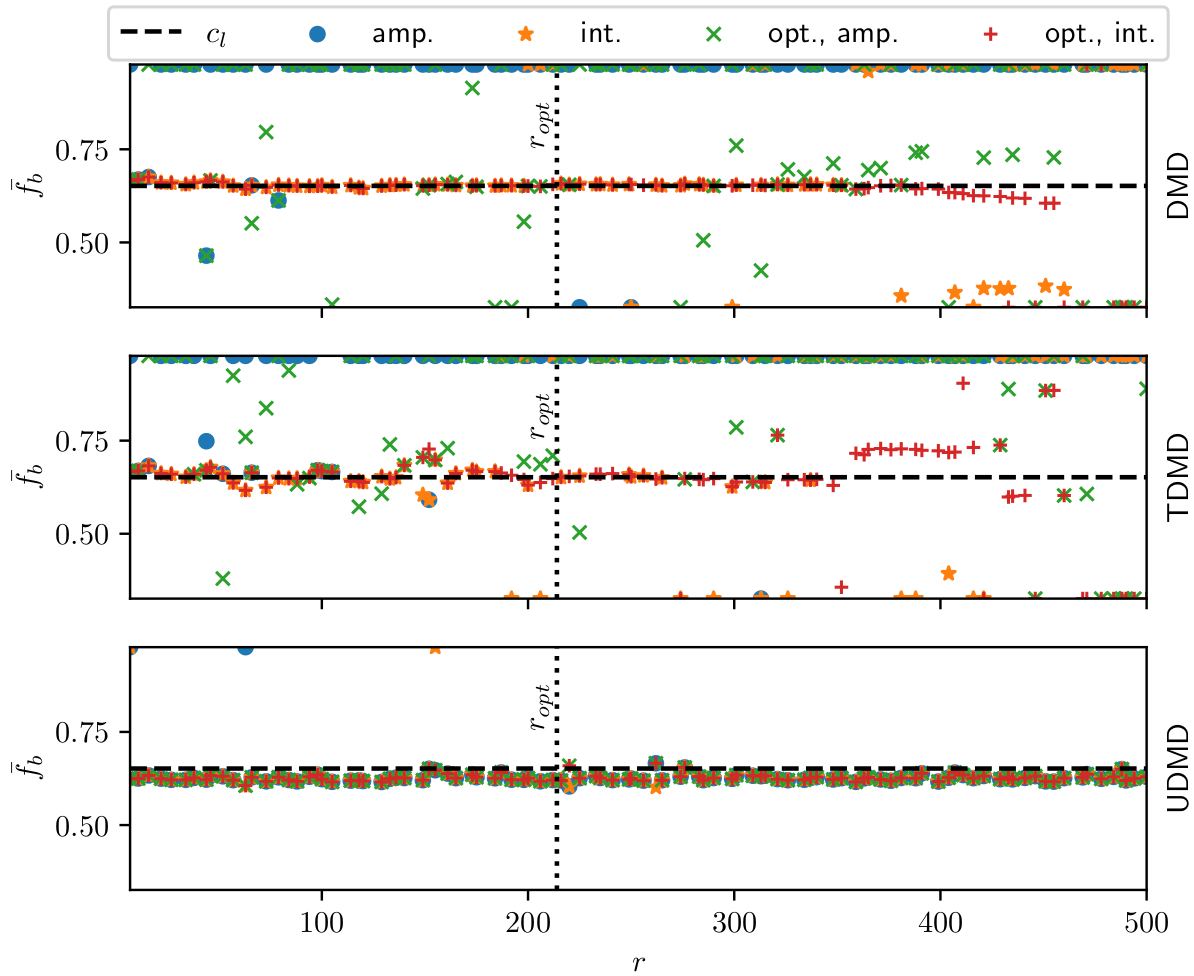Test 2: sensitivity to the sampling rate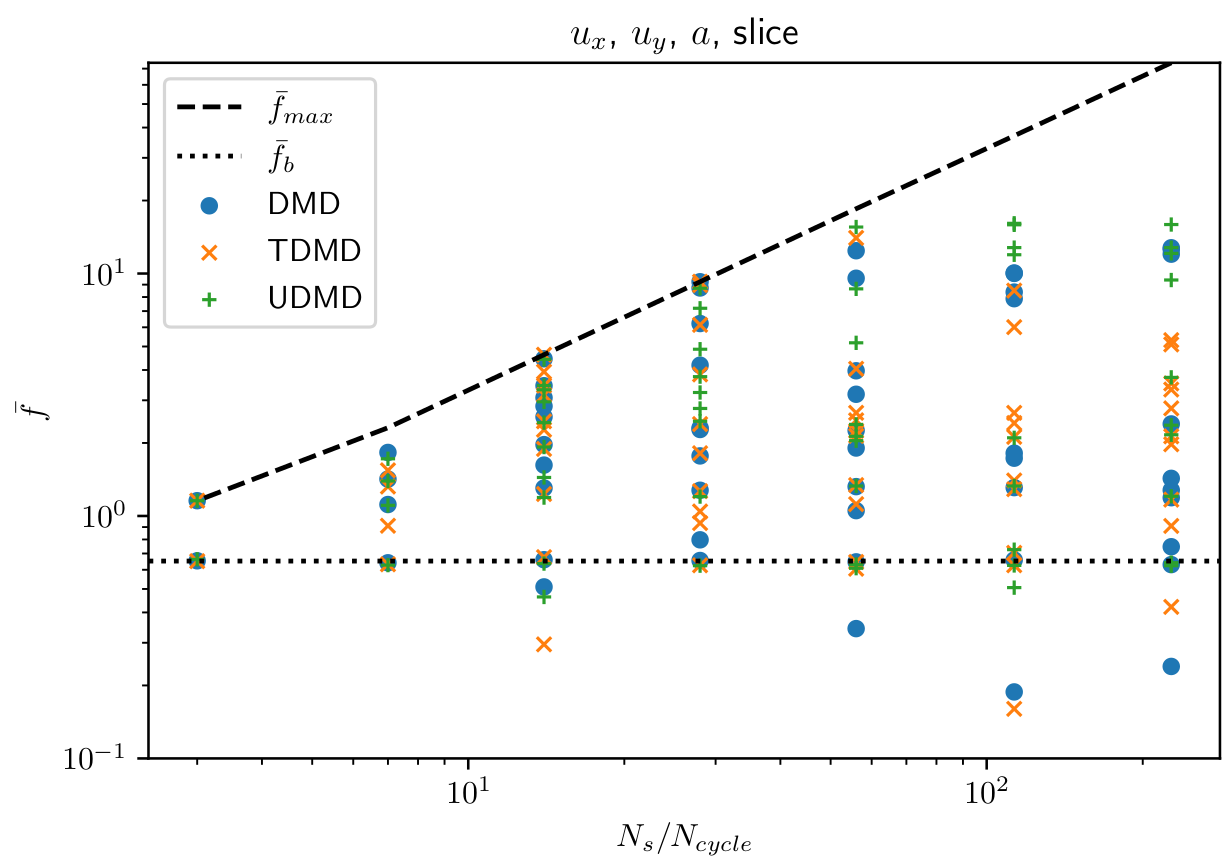Test 3: rank sensitivity of the full spectrum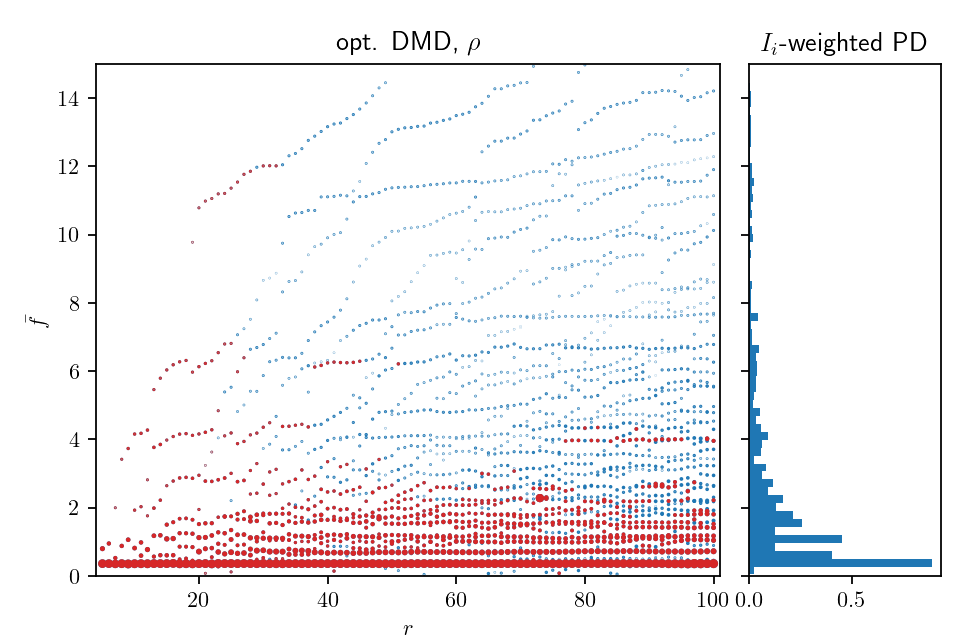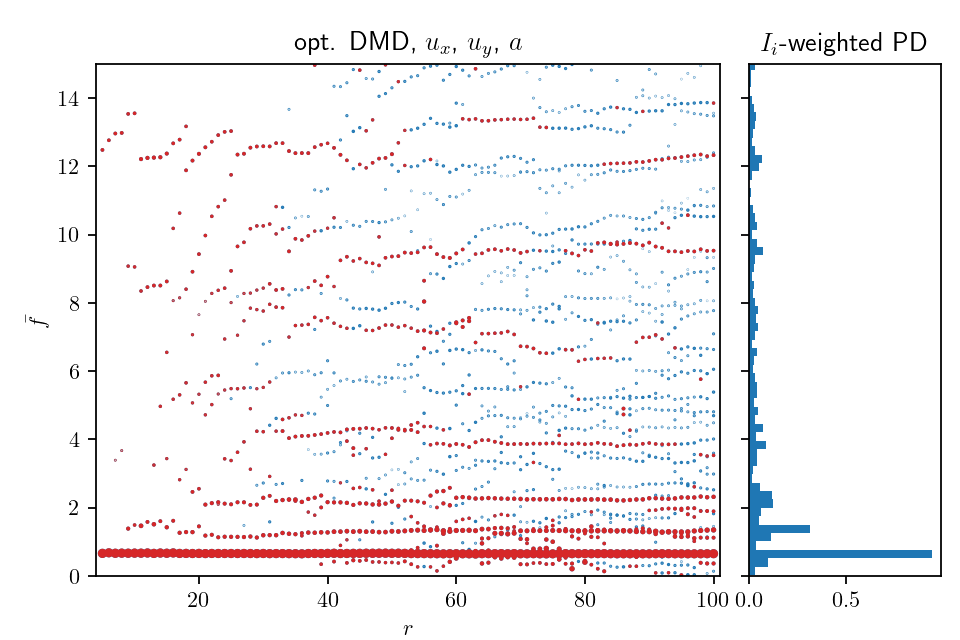Winning configuration:

• physics-based, weighted state vector
• standard operator def.
• optimized amplitudes
• integral selection criterion

## Selected results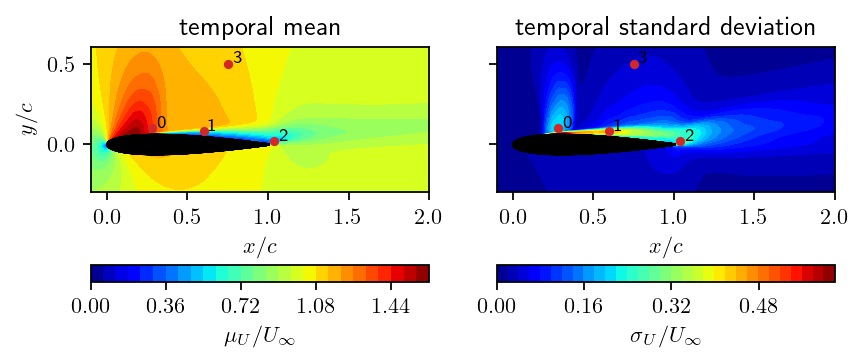Temporal mean, std., and probe locations.PSD based on speed at probe locations.DMD spectrum obtained from slice data.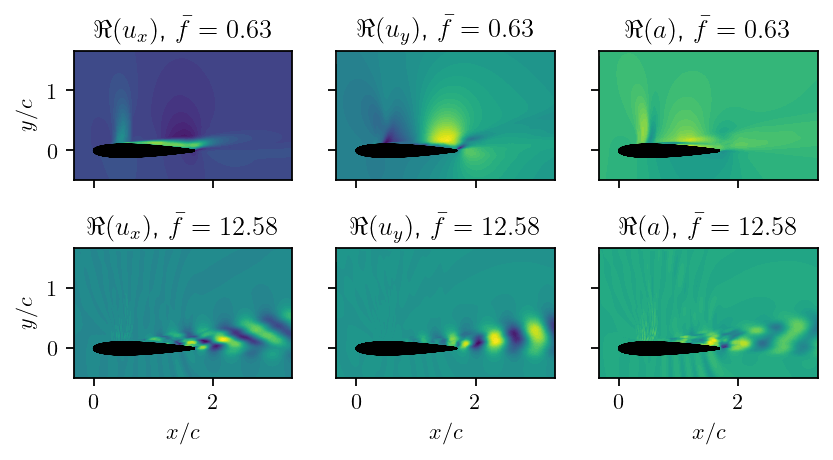Characteristic DMD modes: shock motion and vortex shedding/acoustic waves.

Mode with vortex shedding and acoustic waves.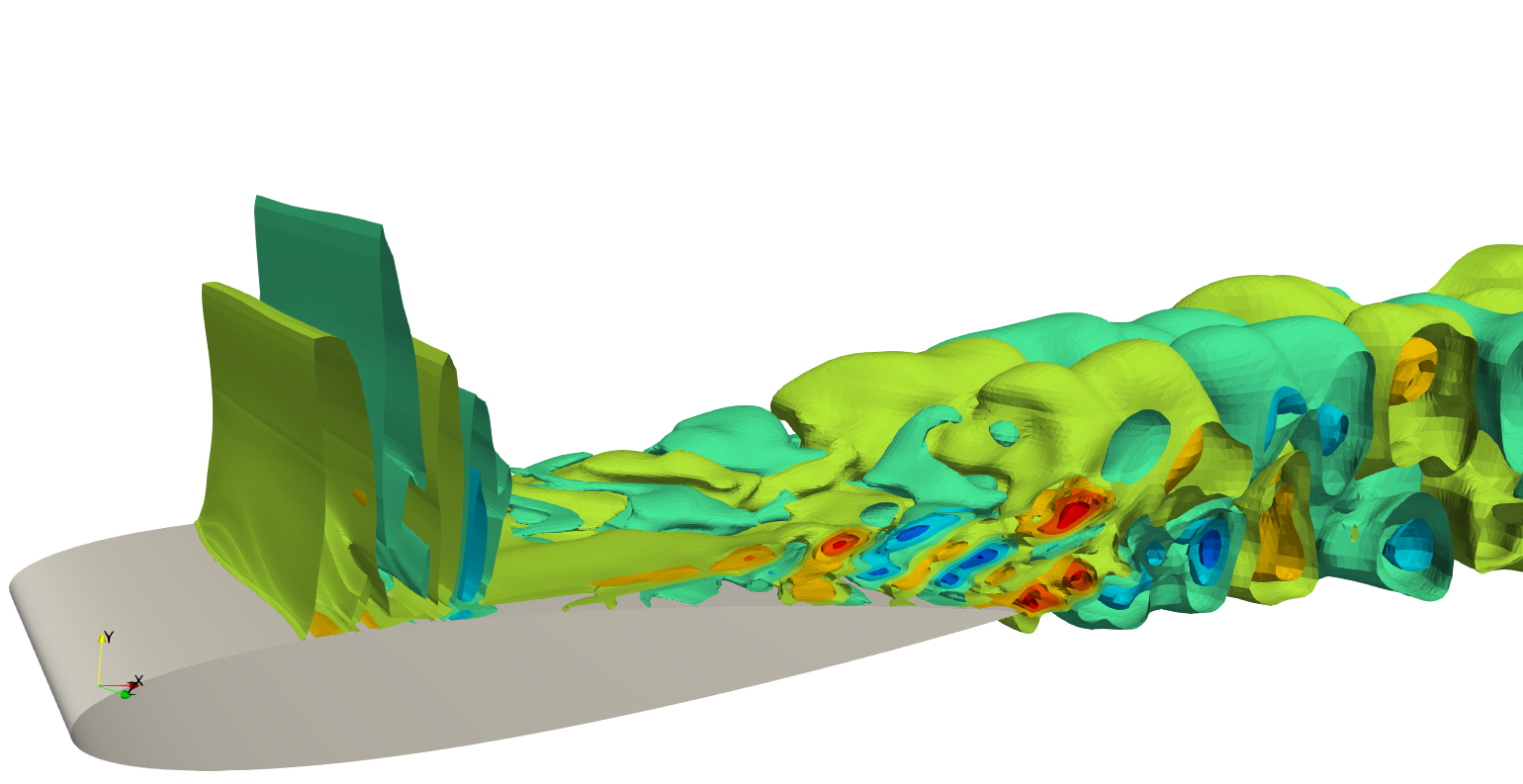Comparable modes and spectra for volume, slice, and surface data.

# THE END

### Thank you for you attention!